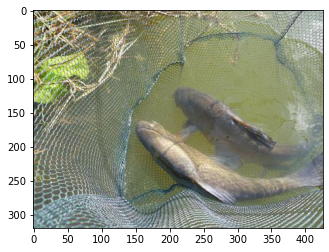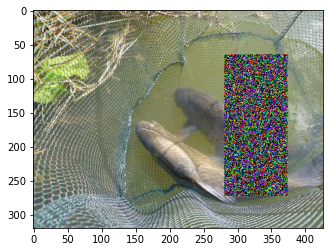From the abstract of the paper:

In training, Random Erasing randomly selects a rectangle region in an image and erases its pixels with random values. In this process, training images with various levels of occlusion are generated, which reduces the risk of over-fitting and makes the model robust to occlusion. Random Erasing is parameter learning free, easy to implement, and can be integrated with most of the CNN-based recognition models.

As seen from image above, this RandomErase data augmentation, randomly selects a region from the input image, erases the existing image in that region and fills the region with random values.

## Training models with RandomErase using timm's training script

To train a model using the RandomErase data augmentation using timm's training script, simply add the --reprob flag with a probability value.

python train.py ../imagenette2-320 --reprob 0.4


Running the above command applies the RandomErase data augmentation to the input images with a probability of 0.4.

## Using RandomErase data augmentation in custom training scripts

Section 1.1 provides an example of using RandomErase data augmentation to train a nueral net using timm's training script. But often you might want to simply just use RandomErase augmentation using your own custom training loop. This section explains how one could achieve that.

The RandomErase data augmentation inside timm is implemented inside RandomErasing class. All we do in the code below, is first create an input image tensor, and visualize it.

from PIL import Image
from timm.data.random_erasing import RandomErasing
from torchvision import transforms
from matplotlib import pyplot as plt

img = Image.open("../../imagenette2-320/train/n01440764/ILSVRC2012_val_00000293.JPEG")
x   = transforms.ToTensor()(img)
plt.imshow(x.permute(1, 2, 0))

<matplotlib.image.AxesImage at 0x7f9ec6879b80>Great, as we can see it is the same image of a "tench" as shown pretty much everywhere inside this documentation. Let's now apply the RandomErasing augmentation and visualize the results.

random_erase = RandomErasing(probability=1, mode='pixel', device='cpu')
plt.imshow(random_erase(x).permute(1, 2, 0))

Clipping input data to the valid range for imshow with RGB data ([0..1] for floats or [0..255] for integers).

<matplotlib.image.AxesImage at 0x7f9ec649e580>As we can see, after applying the RandomErasing data augmentation, a square of random size inside the image has been replaced with random values as mentioned in the paper. Thus, pseudo-code to use RandomErasing in your custom training script would look something like:

from timm.data.random_erasing import RandomErasing

# get input images and convert to torch.tensor
X, y = input_training_batch()
X = convert_to_torch_tensor(X)

# perform RandomErase data augmentation
random_erase = RandomErasing(probability=0.5)

# get augmented batch
X_aug = random_erase(X)

# do something here


## timm's implementation of RandomErase

In this section we will look at the source code of the RandomErasing class inside timm. The complete source code of this class looks like:

class RandomErasing:
""" Randomly selects a rectangle region in an image and erases its pixels.
'Random Erasing Data Augmentation' by Zhong et al.
See https://arxiv.org/pdf/1708.04896.pdf

This variant of RandomErasing is intended to be applied to either a batch
or single image tensor after it has been normalized by dataset mean and std.
Args:
probability: Probability that the Random Erasing operation will be performed.
min_area: Minimum percentage of erased area wrt input image area.
max_area: Maximum percentage of erased area wrt input image area.
min_aspect: Minimum aspect ratio of erased area.
mode: pixel color mode, one of 'const', 'rand', or 'pixel'
'const' - erase block is constant color of 0 for all channels
'rand'  - erase block is same per-channel random (normal) color
'pixel' - erase block is per-pixel random (normal) color
max_count: maximum number of erasing blocks per image, area per box is scaled by count.
per-image count is randomly chosen between 1 and this value.
"""

def __init__(
self,
probability=0.5, min_area=0.02, max_area=1/3, min_aspect=0.3, max_aspect=None,
mode='const', min_count=1, max_count=None, num_splits=0, device='cuda'):
self.probability = probability
self.min_area = min_area
self.max_area = max_area
max_aspect = max_aspect or 1 / min_aspect
self.log_aspect_ratio = (math.log(min_aspect), math.log(max_aspect))
self.min_count = min_count
self.max_count = max_count or min_count
self.num_splits = num_splits
mode = mode.lower()
self.rand_color = False
self.per_pixel = False
if mode == 'rand':
self.rand_color = True  # per block random normal
elif mode == 'pixel':
self.per_pixel = True  # per pixel random normal
else:
assert not mode or mode == 'const'
self.device = device

def _erase(self, img, chan, img_h, img_w, dtype):
if random.random() > self.probability:
return
area = img_h * img_w
count = self.min_count if self.min_count == self.max_count else \
random.randint(self.min_count, self.max_count)
for _ in range(count):
for attempt in range(10):
target_area = random.uniform(self.min_area, self.max_area) * area / count
aspect_ratio = math.exp(random.uniform(*self.log_aspect_ratio))
h = int(round(math.sqrt(target_area * aspect_ratio)))
w = int(round(math.sqrt(target_area / aspect_ratio)))
if w < img_w and h < img_h:
top = random.randint(0, img_h - h)
left = random.randint(0, img_w - w)
img[:, top:top + h, left:left + w] = _get_pixels(
self.per_pixel, self.rand_color, (chan, h, w),
dtype=dtype, device=self.device)
break

def __call__(self, input):
if len(input.size()) == 3:
self._erase(input, *input.size(), input.dtype)
else:
batch_size, chan, img_h, img_w = input.size()
# skip first slice of batch if num_splits is set (for clean portion of samples)
batch_start = batch_size // self.num_splits if self.num_splits > 1 else 0
for i in range(batch_start, batch_size):
self._erase(input[i], chan, img_h, img_w, input.dtype)
return input


All the fun is going inside the _erase method which we will look into next. But in simple words what the above code is doing is that we call this class either passing in a single tensor of size 3 CHW or an input batch of size 4 NCHW. If it's an input batch, and batch is not split similar to Augmix, then we apply the RandomErase data augmentation to the whole batch otherwise we leave the first split as is which becomes the clean split. This splitting of the dataset has already been explained here and here.

Let's now look at the _erase method in detail and understand all the magic.

def _erase(self, img, chan, img_h, img_w, dtype):
if random.random() > self.probability:
return
area = img_h * img_w
count = self.min_count if self.min_count == self.max_count else \
random.randint(self.min_count, self.max_count)
for _ in range(count):
for attempt in range(10):
target_area = random.uniform(self.min_area, self.max_area) * area / count
aspect_ratio = math.exp(random.uniform(*self.log_aspect_ratio))
h = int(round(math.sqrt(target_area * aspect_ratio)))
w = int(round(math.sqrt(target_area / aspect_ratio)))
if w < img_w and h < img_h:
top = random.randint(0, img_h - h)
left = random.randint(0, img_w - w)
img[:, top:top + h, left:left + w] = _get_pixels(
self.per_pixel, self.rand_color, (chan, h, w),
dtype=dtype, device=self.device)
break


The _erase method above accepts an input img (torch.tensor), chan which represents the number of channels in the image and also img_h and img_w which refer to image height and width.

We select a value for count based on self.min_count and self.max_count. The self.min_count has already been set to minimum number of random erase blocks, and self.max_count refers to the maximum number of random erase blocks. Most of the times, both default to 1, that is we only add a single random erase block to the input img.

Next, we select a random target_area and aspect_ratio of the random erase block, and based on these we select the values of the h height and w width of the random erase block.

Finally, we replace the pixels inside the image from location img[:, top:top + h, left:left + w] where top represents a random integer value on the y-axis and left represents a random integer value on the x-axis. The _get_pixels is a function implemented in timm that returns the random values to be filled inside the random erase block depending on the Random Erase mode inside timm.

If mode=='pixel', then, the _get_pixels returns a normal distribution, otherwise a constant value of 0 is filled.